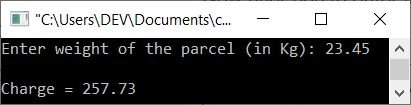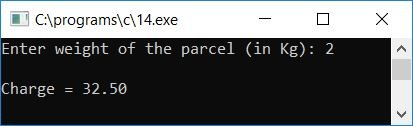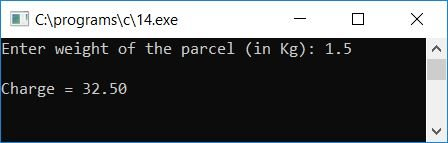# C Program to Compute Charges for Sending Parcels as Per Their Weight

In this post, we will learn about how to create a program in C that will compute and print the charge (that has to be paid by the sender) to send a parcel as per its weight. That is, the program will ask the sender (or user) to enter the weight of the parcel and will calculate and print the charge for sending that parcel (of the given weight).

The program is designed in the following manner:
A post office charges parcel senders according to the weight of the parcel. For each parcel having a weight of 2 kg or less, the charge is 32.50, and for each extra kg, there is an additional charge of 10.50.

```#include<stdio.h>
#include<conio.h>
int main()
{
float weight, initCharge=32.50, perCharge=10.50, tempWeight, addCharge, totalCharge;
printf("Enter weight of the parcel (in Kg): ");
scanf("%f", &weight);
printf("\n");
if(weight<=2)
printf("Charge = %0.2f", initCharge);
else
{
tempWeight = weight-2;
printf("Charge = %0.2f", totalCharge);
}
getch();
return 0;
}```

As the above program was written in the Code::Blocks IDE, here is the sample run after a successful build and run. This is the first snapshot:The user must now enter the weight of the parcel to determine the total charge that must be paid. Here is the second snapshot of the same sample run:If the weight of the parcel is less than or equal to 2, then there is only 32.50 that has to be paid by the sender. Let's take a look at another sample run:Let's take another sample run, as given below. This time the user has entered the parcel weight as 1.5 kg:Below are some of the main steps used in the above program:

• Take any two variables, say initCharge and perCharge. Set initCharge to 32.50 (charge for parcels weighing less than or equal to 2 kg) and perCharge to 10.50 (charge for each additional kg of parcel weight).
• At runtime, get the weight of the parcel from the user.
• Check whether the given weight is less than or equal to 2 or not.
• If it is, then just print the value of initCharge as output that holds the charge value of a parcel with a weight less than or equal to 2 kg.
• Otherwise, if the weight of the parcel is greater than 2 kg, then follow the steps given below.
• Because the first 2 kg of parcel weight is 32.50,Therefore, we have to subtract the original weight by 2 and then compute the charge as per the given rate, which is \$10.50 per extra kilogram. And then add the calculated charge to 32.50; after this, you will get the final charge that has to be paid by the parcel sender to send the parcel.
• For example, if the user has entered the weight of the parcel as 10 kg, Because the value 10 is greater than 2, the program flow enters the else block.
• Inside the else block, weight-2 or 10-2 or 8 is initialized to tempWeight, then tempWeight * perCharge or 8 * 10.50 or 84 is initialized to addCharge, and then addCharge + initCharge or 84 + 32.50 or 116.5 is initialized to totalCharge
• Print the value of totalCharge as output, which will be the final charge (to be paid) to send the parcel.

C Quiz

« Previous Program Next Program »